# Call by Value and Call by Reference in JavaScript

## Call by Value and Call by Reference in JavaScript

In this article, I am going to discuss Call by Value and Call by Reference in JavaScript with Examples. Please read our previous article where we discussed JavaScript Object Properties with Special Characters with Examples.

##### Call by Value and Call by Reference in JavaScript

This concept is important to understand in order to learn any programming language and its memory management system, and how they handle variables defined to store value in the code. As we have seen and learned JavaScript is a TypeLess language. It means variables don’t have a type, but the values they hold do have a type.

A Variable is a vessel to store a value whereas a variable reference is an actual address/location in the memory where the variable is stored. JavaScript provides 2 categories of data types: Primitives and Objects. JavaScript has six different types:

1. number
2. string
3. boolean
4. null
5. undefined and
6. object

Primitives are value types and Object type is a reference type in JavaScript. The Value types are stored on the stack and reference types are stored on the heap.

The object is the only Object type. It is copied by reference. number, string, boolean, null, and undefined are primitive types and copied by value. The primitive types are number, string, boolean, null, and undefined. All the other types are of object type Including arrays, functions, dates, custom types, etc. For Example:

console.log(typeof new Object() === typeof new Array()); //true
console.log(typeof new Object() === typeof new Date()); //true
console.log(typeof new Array() === typeof new Date()); //true

##### Difference between comparing values type and reference types in JavaScript:

When we use the strict comparison operator ===, 2 variables having value types are equal. If they have the same value. For Example:

const valueType1 = 1;
const valueTypeCopy = 1;
console.log(valueType1 === valueTypeCopy); // true
console.log(valueType1 === 1); // true
console.log(valueType1 === valueType1); // true

Both valueType1 and valueTypeCopy have the same value1. The operator === evaluates to true if both the operands are 1, no matter where the value is taken from: for example, as expression 2-1. When having reference types, the comparison operator === behaves differently. 2 references are equal if they are both referencing the same object. For Example:

const array1 = ;
const array2 = ;
console.log(array1 === array2); // false
console.log(array1 === ); // false
const array11 = array1;
console.log(array1 === array11); // true
console.log(array1 === array1); // true

Arrays array1 and array2 share the same reference array structure, yet array1 === array2 evaluates to false since arrays array1 and array2 refer to different array objects. Comparison operators return true only when references type point to the same object. For example, array1 === array11, and array1 === array1.

All data types derive from objects. Their type is an object. JavaScript provides a feature to combine this primitive datatype into compound data types such as array and object. both array and objects are ways of grouping/ combining pieces of data altogether into the collection. For Example:

var daysOfWeek = [“Monday”, “Tuesday”, “Wednesday”, “Thursday”, “Friday”, “Saturday”, “Sunday”]; //array of string collection
var number = [1, 2, 3, 4, 5, 6, 7] //array of number collection

What we discussed above is given in the below example.

##### Example1: JavaScript Objects Comparing Reference, and Primitive Types
```<html>
<title>JavaScript Objects comparing reference, and primitive types example1</title>
<body>
<script>
//reference type comparison
console.log(typeof new Object() === typeof new Array()); //true as it’s an object
console.log(typeof new Object() === typeof new Date()); //true
console.log(typeof new Array() === typeof new Date()); //true

//comparing value type
console.log("comparing value type");
const valueType1 = 1;
const valueTypeCopy = 1;
console.log(valueType1 === valueTypeCopy); // true
console.log(valueType1 === 1); // true
console.log(valueType1 === valueType1); // true

//comparing reference type
console.log("comparing reference type");
const array1 = ;
const array2 = ;
console.log(array1 === array2); // false
console.log(array1 === ); // false
const array11 = array1;
console.log(array1 === array11); // true
console.log(array1 === array1); // true

</script>
</body>
</html>
```
###### Output: Press F12 and go to the Console section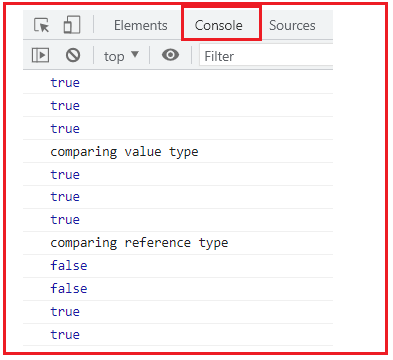##### Primitive Types in JavaScript

Primitive types are passed by value. When passed as an argument. The new memory is allocated in the stack. The value is copied into the new memory. The value in the new memory is passed. Primitive types are initialized with type literals. For Example:

var number = 5;
var text = ‘Hello!’;

Primitive types have an object-type(reference-type) wrapper which means each primitive type has a corresponding object type wraps around the primitive types such as int, or number. It temporarily wraps the primitive type into the object type, then executes the statement, and later unboxes the primitive types. The object is temporary means it is last for a short period of time, and we normally don’t get to see this whole scenario. That means if we try to set a property on a temporary object wrapped around the primitive type, it does not persist. For Example:

var number = 100;
number.strValue = “Hundred in words”;
console.log(number.strValue); //undefined

In Pass by Value, the value of the variables is passed as function arguments/parameters. Any changes done in the arguments/parameters do not affect/impact the value of the variables passed from outside the function. We will try to understand how value types or primitive types work.

// strings
let x = ‘JavaScript’
let y = ‘JavaScript’
console.log(x === y) // return true

Reason? The answer is easy because JavaScript checks the value of the variables if they are equal or not.

let z = x;
console.log(z === x)//return true
x = ‘language’
console.log(z)// return JavaScript

In the below example, we have assigned string values to two variables fname = ‘Shagufta’ and lname = ‘Angel’. Created an object using its value and change the value of the variables. Each object has its own value. What we discussed above is given in the below example.

##### Example2: JavaScript Objects, Primitive Types
```<html>
<title>JavaScript Objects, primitive types example2</title>
<body>
<script>
// Primitive types have a reference type wrapper
var number = 5; // holds a primitive value of 5
var numberObj = new Number(5); // holds a reference value of 5

console.log('(number == numberObj) = ' + (number == numberObj));
console.log('(number === numberObj) = ' + (number === numberObj));
console.log('typeof number = ' + typeof number);
console.log('typeof numberObj = ' + typeof numberObj);
console.log('(typeof number === typeof numberObj) = ' + (typeof number === typeof numberObj));

//Primitive types are passed by value
console.log("-------------------");

var fname = 'Shagufta';
var lname = 'Angel';
var person = {
firstName: fname,
lastName: lname,
toString: function personToString() {
return this.firstName + " " + this.lastName
}
};

console.log(person);
console.log('Changing the last name to Doe');
lname = 'Doe';
console.log(person) // still "Angel"
</script>
</body>
</html>
```
###### Output: Press F12 and go to the Console section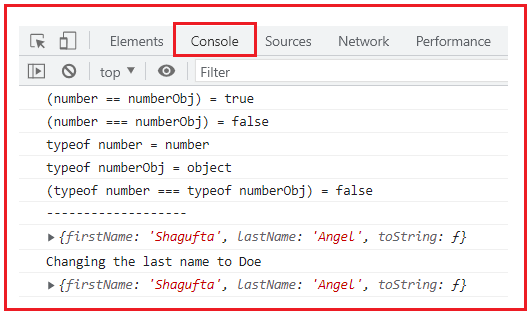##### Example3: JavaScript Objects, JavaScript Pass by Value
```<html>
<title>JavaScript Objects, JavaScript Pass by Value example3</title>
<body>
<script>
function callByValue(name) {
name = 'Kathy';
console.log(name);
}

var name = 'JavaScript';
console.log(name); //JavaScript
callByValue(name); //Kathy
console.log(name); //JavaScript

</script>
</body>
</html>
```
###### Output: Press F12 and go to the Console section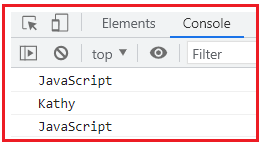In the above example, the value of the variable name is not changed, because it is passed as a value.

##### Object Types in JavaScript

Object types are passed by reference. The object is the only object type. When passed to a function, the value is not copied, but rather, a reference of it is passed.

JavaScript passed objects as arguments, which means when JavaScript objects are passed as arguments to a function/method, they are passed as reference, not by value. This means that the object itself is accessible not its copy/duplicate and mutable that can be changed inside the function/method.

In Pass by Reference, the reference/ address of the variables is passed as function arguments/parameters. Any changes done in the arguments affect the actual value of the variables passed from outside the function.

When we create an object, we give it a reference. If two variables hold the same reference, any changes to the object will affect both variables. In JavaScript Objects, functions and Arrays are passed by Reference. We will try to understand how reference or object types work.

// Objects
let fruit = { name: ‘Mango’ }
let fruitJuice = { name: ‘Mango’ }
console.log(fruit === fruitJuice) // return false

Reason? The answer is false because the values of both objects fruit and fruitJuice are the same, but the JavaScript engine compares the reference of two variables which is different hence it is false.

let anotherFruit = fruit;
console.log(anotherFruit === fruit)//return true
fruit.name = ‘apple’
console.log(anotherFruit)// {name: ‘apple’}

What we discussed above is given in the below example.

##### Example4: JavaScript Objects, Reference Types
```<html>
<title>JavaScript Objects, reference types example4</title>
<body>
<script>
function printStudentDetails(student) {
console.log('<strong>' + student.name + '</strong>');
for (var i in marks) {
console.log('<strong>' + marks[i].subject + '</strong>' + ' : ' + marks[i].score);
}
console.log('--------------------------');
}

var marks = [
{ subject: 'JavaScript', score: 5.50 },
{ subject: 'OOP', score: 5.00 },
{ subject: 'Java', score: 6.00 },
{ subject: 'C#', score: 4.00 }];

var student = { name: 'Maria Anthony', marks: marks };
printStudentDetails(student);
marks.score = 5.50;
printStudentDetails(student);
</script>
</body>
</html>
```
###### Output: Press F12 and go to the Console section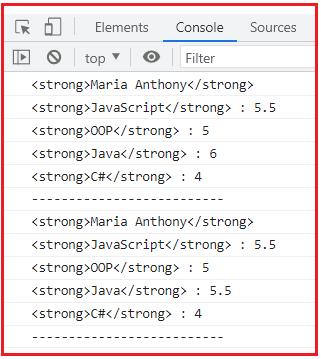##### Example5: JavaScript Objects, JavaScript Passing Objects as Arguments
```<html>
<title>JavaScript Objects, JavaScript passing objects as arguments example5</title>
<body>
<script>

const originMarks = 80;
const OriginStudent = { name: 'Angel' };

const changeTheDetails = (marks, obj) => {
marks = 95;
obj.name = 'John';
};

changeTheDetails(originMarks, OriginStudent);

// Will output 80 since integers are passed by value.
console.log(originMarks);

// Will output 'John' since objects are passed
// by reference and are therefore mutable.
console.log(OriginStudent.name);
</script>
</body>
</html>
```
###### Output: Press F12 and go to the Console section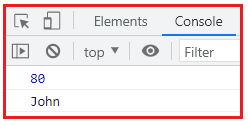##### Example6: JavaScript Objects, JavaScript Pass by Reference
```<html>
<title>JavaScript Objects, JavaScript Pass by Reference example6</title>
<body>
<script>
function callByReference(object) {
object.name = 'Kathy';
console.log(object);
}

var obj = { name: 'JavaScript' };
console.log(obj); //{ name: 'JavaScript' };
callByReference(obj); //{ name: 'Kathy' };
console.log(obj); //{ name: 'Kathy' };

</script>
</body>
</html>
```
###### Output: Press F12 and go to the Console section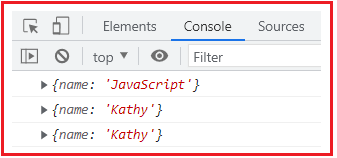In the above example, since objects are passed as reference, hence the value of the object property name is changed.

##### Object Cloning in JavaScript

Shared object– two variables holding the same object.

##### Example7: JavaScript Objects, object cloning by the shared object
```<html>
<title>JavaScript Objects, object cloning by shared object example7</title>
<body>
<script>

var angel = { name: 'Angel', age: 20 };
var maria = angel;
maria.name = 'Maria';
console.log('maria object: ', maria);
console.log('angel object: ', angel);
</script>
</body>
</html>
```
###### Output: Press F12 and go to the Console section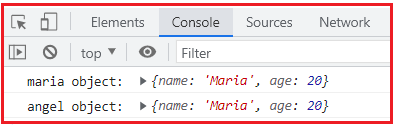Cloned objects– two variables holding separate objects.

##### Example8: JavaScript Objects, object cloning by cloned objects
```<html>
<title>JavaScript Objects, object cloning by cloned objects example8</title>
<body>
<script>

var angel = { name: 'Angel', age: 20 };
var maria = JSON.parse(JSON.stringify(angel));
maria.name = 'Maria';
console.log('maria object: ', maria);
console.log('angel object: ', angel);
</script>
</body>
</html>
```
###### Output: Press F12 and go to the Console section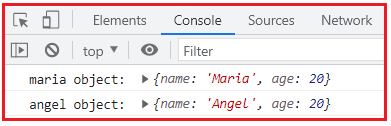##### Difference between Call by Value and Call by Reference
 Call by Value Call by Reference Definition While invoking a function/method when we pass values by copying the variables. It is called Call by Values. While invoking a function/method, rather than copying the values of variables, the actual address of the variables is used it is called Call by Reference. Arguments/Parameters In this method, a copy of the variable is passed. In this method, the variable itself is passed. Impact/Affect Any changes made in the copy of the variable never modify the value of the variable located outside the function. Any changes made in the variable impact/affect the value of the variable located outside the function. Memory Location Actual and formal arguments/parameters will be created in different memory locations/addresses. Actual and formal arguments/parameters will be created in the same memory location/address.

In the next article, I am going to discuss JavaScript Objects are Mutable with Examples. Here, in this article, I try to explain Call by Value and Call by Reference in JavaScript with Examples and I hope you enjoy this Call by Value and Call by Reference in JavaScript with Examples article.